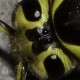Jonathan Mee -4 years ago 46
C Question

# Cross Platform Support for sprintf's Format '-Flag

The Single UNIX Specification Version 2 specifies the

`sprintf`
's format
`'`
-flag behavior as:

The integer portion of the result of a decimal conversion (
`%i`
,
`%d`
,
`%u`
,
`%f`
,
`%g`
or
`%G`
) will be formatted with thousands' grouping characters

I can't find the format
`'`
-flag in the or the specifications. g++ even warns:

ISO C++11 does not support the
`'`
`printf`
flag

The flag is not recognized to even warn about in Visual C;
`printf("%'d", foo)`
outputs
:

'd

I'd like to be able to write C-standard compliant code that uses the behavior of the format
`'`
-flag. Thus the answer I'm looking for one of the following:

1. C-Standard specification of the format
`'`
-flag

2. A cross platform compatible extrapolation of gcc's format
`'`
-flag

3. Demonstration that a cross platform extrapolation is not possibleJerry Coffin
Answer Source

Standard C doesn't provide the formatting capability directly, but it does provide the ability to retrieve a...specification of what the formatting should be, on a locale-specific basis. So, it's up to you to retrieve the locale's specification of proper formatting, then put it to use to format your data (but even then, it's somewhat non-trivial). For example, here's a version for formatting `long` data:

``````#include <stdlib.h>
#include <locale.h>
#include <string.h>
#include <limits.h>

static int next_group(char const **grouping) {
if ((*grouping) == CHAR_MAX)
return 0;
if ((*grouping) != '\0')
++*grouping;
return **grouping;
}

size_t commafmt(char   *buf,            /* Buffer for formatted string  */
int     bufsize,        /* Size of buffer               */
long    N)              /* Number to convert            */
{
int i;
int len = 1;
int posn = 1;
int sign = 1;
char *ptr = buf + bufsize - 1;

struct lconv *fmt_info = localeconv();
char const *tsep = fmt_info->thousands_sep;
char const *group = fmt_info->grouping;
char const *neg = fmt_info->negative_sign;
size_t sep_len = strlen(tsep);
size_t group_len = strlen(group);
size_t neg_len = strlen(neg);
int places = (int)*group;

if (bufsize < 2)
{
ABORT:
*buf = '\0';
return 0;
}

*ptr-- = '\0';
--bufsize;
if (N < 0L)
{
sign = -1;
N = -N;
}

for ( ; len <= bufsize; ++len, ++posn)
{
*ptr-- = (char)((N % 10L) + '0');
if (0L == (N /= 10L))
break;
if (places && (0 == (posn % places)))
{
places = next_group(&group);
for (int i=sep_len; i>0; i--) {
*ptr-- = tsep[i-1];
if (++len >= bufsize)
goto ABORT;
}
}
if (len >= bufsize)
goto ABORT;
}

if (sign < 0)
{
if (len >= bufsize)
goto ABORT;
for (int i=neg_len; i>0; i--) {
*ptr-- = neg[i-1];
if (++len >= bufsize)
goto ABORT;
}
}

memmove(buf, ++ptr, len + 1);
return (size_t)len;
}

#ifdef TEST
#include <stdio.h>

#define elements(x) (sizeof(x)/sizeof(x))

void show(long i) {
char buffer;

commafmt(buffer, sizeof(buffer), i);
printf("%s\n", buffer);
commafmt(buffer, sizeof(buffer), -i);
printf("%s\n", buffer);
}

int main() {

long inputs[] = {1, 12, 123, 1234, 12345, 123456, 1234567, 12345678 };

for (int i=0; i<elements(inputs); i++) {
setlocale(LC_ALL, "");
show(inputs[i]);
}
return 0;
}

#endif
``````

This does have a bug (but one I'd consider fairly minor). On two's complement hardware, it won't convert the most-negative number correctly, because it attempts to convert a negative number to its equivalent positive number with `N = -N;` In two's complement, the maximally negative number doesn't have a corresponding positive number, unless you promote it to a larger type. One way to get around this is by promoting the number the corresponding unsigned type (but it's is somewhat non-trivial).

Implementing the same for other integer types is fairly trivial. For floating point types is a bit more work. Converting floating point types (even without formatting) correctly is enough more work that for them, I'd at least consider using something like `sprintf` to do the conversion, then inserting the formatting into the string that produced.

Recommended from our users: Dynamic Network Monitoring from WhatsUp Gold from IPSwitch. Free Download
Latest added